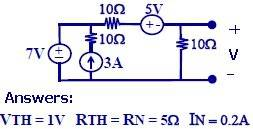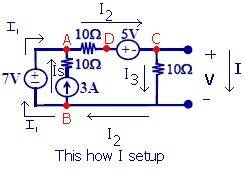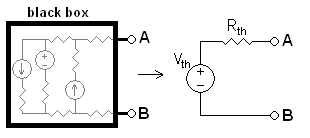# Thevenin Circuit Question

• EngineeringI don't know which node to take as zero voltage. Either C or B or both.
Finding Out I-V characteristic of +V-. Considering Vb = 0.

ASSUMING V IS SOME LOAD

I = I2 - I3
I = ((Va - Vd)/10) - ((Vc - Vb)/10)
I = ((7 - 5)/10) - ((Vc - 0)/10)
I = 0.2 - Vc/10

Where Vc - Vb = V
Considered Vb = 0
So Vc = V

Therefore;
I = 0.2 -V/10

However the Correct Answer is:

I = 0.2 - V/5
---------------------------------------------------------------------
I am getting Correct current (0.2A when V = 0)
But wrong Rth (Thevenin Resistance) as 10 ohms.

Can someone point me where Iam doing wrong in the method IAM using for
calculating I-v characteristic.
I know other shortcuts methods that give me correct answer but I want
to know what mistake I am doing and how to correct it.
----------------------------------------------------------------------

Last edited:

## Answers and Replies

Defennder
Homework Helper
You need to make clear where the current I is flowing. Are you trying to find the open circuit thevenin voltage or the short-circuit current? Because in the diagram, I = I2 - I3 doesn't appear anywhere in both cases. In the open circuit case, I2=I3; in the short circuit case I=I2 since the 10 ohm resistor is shorted.

Lets assume V is some load in the picture above.

Defennder
Homework Helper
Well how are you calculating Rth from the above?

Last edited:
I don't know which node to take as zero voltage.
It doesn't matter very much, just calculate the voltage in the output open for the thevenin equivalent and the current in the output shorcircuited. To solve the circuit you have to have to select a zero voltage node, but you can put it where you want, I like to put in the minus of the output.
ASSUMING V IS SOME LOAD
I don't understand this sentence.

The easiest way to solve this problems is to use the superposition theorem to calculate the thevenin or norton source:
http://en.wikipedia.org/wiki/Superposition_theorem
Then calculate the equivalent resitance from the output placing an open circuit, instead the current sources, and a shorcircuit, instead the voltage sources. In this problem is clear that is the parallel between the resistance in series with the voltage sources, hence 5ohms.
hope this help

Well how are you calculating Rth from the above?

Using general form of Thevenin Circuit (according to Circuit Diagram):I = (-V/Rth) + (Vth/Rth)

When I derive my I-V characteristics I compare the two equations.
Since what I calculated is:
I = -V/10 + 0.2

So
Rth = 10
Vth/Rth = I (when V= 0, intersection) = In = 0.2

This is long way of doing and I have been having correct answers for all thevenin circuits I attempted, except this one. I know the shortcuts of getting answers by making open circuits and short circuits. But thats not I am asking here.
I need to find error in my reasoning for I-V characteristic i did at thread#1

Defennder
Homework Helper
I don't know which node to take as zero voltage. Either C or B or both
No you cannot assume both are at ground potential, otherwise you are already implicitly assuming that V is a short-circuited load.
I = I2 - I3
I = ((Va - Vd)/10) - ((Vc - Vb)/10)
I = ((7 - 5)/10) - ((Vc - 0)/10)
I = 0.2 - Vc/10
Where Vc - Vb = V
Considered Vb = 0
So Vc = V
Your error is here. Vd cannot be 5 unless Vc=Vb=0, which is an invalid assumption unless it is a short-circuit. Instead, let Vd = V+5. That should fix it.

I don't see how useful your method can be. It clearly is a lot longer than the standard textbook treatment of Thevenin equivalent. And prone to mistakes as well. You could have simply calculated Rth by finding equivalent resistance after zeroing all the sources.

uart
Science Advisor
Defender beat me to it but let me echo his comments, there are several different ways you can handle Thevinens all of which are much easier than your method. Personally I could solve that circuit by inspection in under 20 seconds.

Anyway here is your method "fixed".
I = I2 - I3
I = ((Va - Vd)/10) - ((Vc - Vb)/10)
*** I = ((7 - 5)/10) - ((Vc - 0)/10) *** eqn in error
*** I = 0.2 - Vc/10

I = (7 - (Vc+5))/10 - Vc/10
I = (7-5)/10 -V/10 -V/10
I = 1/5 - V/5

If I assume Vc = 0, then Vd = 5. And so Vb cannot be equal to zero.
Vab = 7v.
Va = 7 + Vb

I = I2 - I3
I = (Vad/10) - (Vcb/10)
I = Va/10 -5/10 +Vb/10
I = (7 + Vb)/10 -1/2 +Vb/10
I = Vb/5 + 7/10 -1/2
I = Vb/5 + 1/5

where Vb = -V
So
I = -V/5 + 1/5

Thanks! it also works the other way :)
-------------------------------------------------
I have two more questions

1) Is it always true that in any circuit there is a point of zero voltage?
2) Can someone show me a little proof on the method of calculating Rth fast? (The method: open circuit for Current source and Short circuit for Voltage source)

Thanks
-------------------------------------------------

1) Is it always true that in any circuit there is a point of zero voltage?
I suppose that you mean voltage V in the output, well I would say that when de load resitance is zero, there is no voltage, and the current is the Norton current. As well if the load in infinite, open output, the voltage is the thevenin voltage. But I don't understand very well your question, because this explanation are a bit straightforward, and maybe you want to say something more complicated.

Can someone show me a little proof on the method of calculating Rth fast? (The method: open circuit for Current source and Short circuit for Voltage source)
Well in this case, try to draw the circuit shorting the voltage sources and opening the current sources, do you see that the network is two resistance of 10ohms in parallel? then, you got it! It's very easy, although sometimes is better to plot it, with practice inspection will be enough.
Hope this help